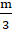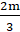Daily Practice Problems
Class 12 Physics
Dual Nature of Radiation and MatterQuestion 1:

An electron, an alpha-particle, and a proton have the same kinetic energy. Which of these particles has the shortest de Broglie wavelength?

(a) electron

(b) proton

(d) Cannot say

Question 2:

The stopping potential (V0) depends on

(a) the frequency of incident light

(b) the nature of the emitter material.

(c) for a given frequency of incident light, it is independent of its intensity

(d) all of the above

Question 3:

In Davison- German experiment, an electron beam is incident on a crystal. The reflected beam consists of

(a) photons

(b) protons

(c) X-rays

(d) electrons

Question 4:

What is the de Broglie wavelength of an electron accelerated through a Potential difference of V volts

(a) λ = 1.227/Vnm

(b) λ = 1.227/V nm

(c) λ = 1.227√Vnm

(d) None

Question 5:

An electron and proton have the same de-Broglie wavelength. Then K.E. of the electron is

(a) zero

(b) infinity

(c) equal to K.E. of the proton

(d) greater than K.E. of proton

Question 6:

Photoelectric effect shows

(a) wave nature of electrons

(b) particle nature of light

(c) both (a) &(b)

(d) none of these

Question 7:

A photocell is a device which

(a) converts light into electricity

(b) converts electricity into light

(c) stores light

(d) stores electricity

Question 8:

The dimension of work function is ----

(a) [ML2 T–2]

(b) [ML2 T–1]

(c) [ML 2 T–3A–1]

(d) None

Question 9:

If the kinetic energy of the particle is increased by 16 times, the percentage change in the de-Broglie wavelength of the particle is

(a) 25%

(b) 75%

(c) 60%

(d) 50%

Question 10:

The de-Broglie wavelength of an electron in the 1st Bohr orbit is

(b) equal to twice the circumference of the 1st orbit

(c) equal to half the circumference of the 1st orbit

(d) equal to one-fourth the circumference of the two orbit

Question 11:

The maximum kinetic energy of a photoelectron is 3 eV. What is the stopping potential?

Question 12:

Why are alkali metals most suited for photoelectric emission?

Question 13:

Find the de-Broglie wavelengths of (a) a 46-g golf ball with a velocity of 30 m/s and (b) an electron with a velocity of 107 m/s.

Question 14:

The work function of caesium is 2.14 eV. Find (a) the threshold frequency for caesium, and (b) the wavelength of the incident light if the photocurrent is brought to zero by a stopping potential of 0.60 V.

Question 15:

A nucleus of mass M initially at rest splits into two fragments of massesand. Find the ratio of de-Broglie wavelength of the two fragments.

Question 16:

What is the de Broglie wavelength associated with an electron, accelerated through a potential difference of 100 volts?

Question 17:

Estimate the ratio of the wavelengths associated with the electron orbiting around the nucleus in the ground and first excited states of a hydrogen atom.

Question 18:

Two monochromatic beams A and B of equal intensity l hit a screen. The number of photons hitting the screen by beam A is twice that by beam B. Then what inference can you make about their frequencies?

Question 19:

Find the de-Broglie wavelength of neutron at 27o C. Given the Boltzmann constant 1.38× 10-23 Jmolecule-1k-1, h = 6.63× 10-34Js , mass of neutron 1.66× 10-23 kg.

Question 20:

A proton and an alpha particle, both initially at rest, are (suitably) accelerated so as to have the same kinetic energy. What is the ration of their de-Broglie wavelengths?

************